##### Question

In: Statistics and Probability

# A population of values has a normal distribution with μ = 96.2 μ = 96.2 and...

A population of values has a normal distribution with μ = 96.2 μ = 96.2 and σ = 15 σ = 15 . You intend to draw a random sample of size n = 92 n = 92 .

Find the probability that a single randomly selected value is between 94 and 96.5. P(94 < X < 96.5) =

Find the probability that a sample of size n = 92 n = 92 is randomly selected with a mean between 94 and 96.5. P(94 < M < 96.5) =

## Solutions

##### Expert Solution

Solution :

Given that ,

mean =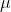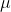= 96.2

standard deviation =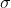= 15

P(94< x <96.5 ) = P[(94 - 96.2) / 15< (x -) /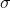< (96.5 - 96.2 ) /15 )]

= P( -0.15< Z <0.02 )

= P(Z <0.02 ) - P(Z < -0.15)

Using z table

= 0.5080 - 0.4404

probability= 0.0676

b.

Solution :

Given that ,

mean == 96.2

standard deviation == 15

n = 92

sample distribution of sample mean is ,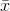== 96.2

sampling distribution of standard deviation=/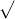n = 15/92=1.56= 1.56

P(94 < M < 96.5) = = P[(94 - 96.2) /1.56 < (-) /< (96.5 - 96.2) / 1.56)]

= P( -1.41< Z <0.19 )

= P(Z < 0.19) - P(Z <-1.41 )

Using z table

=0.5753 - 0.0793

=0.4960

probability= 0.4960

## Related Solutions

##### A population of values has a normal distribution with μ = 118.4 μ = 118.4 and...
A population of values has a normal distribution with μ = 118.4 μ = 118.4 and σ = 87.7 σ = 87.7 . You intend to draw a random sample of size n = 24 n = 24 . Find the probability that a single randomly selected value is less than 62.9. P(X < 62.9) = ______ Find the probability that a sample of size n = 24 n = 24 is randomly selected with a mean less than 62.9....
##### A population of values has a normal distribution with μ = 110.6 μ = 110.6 and...
A population of values has a normal distribution with μ = 110.6 μ = 110.6 and σ = 74 σ = 74 . You intend to draw a random sample of size n = 157 n = 157 . Find P68, which is the score separating the bottom 68% scores from the top 32% scores. P68 (for single values) = Find P68, which is the mean separating the bottom 68% means from the top 32% means. P68 (for sample means)...
##### A population of values has a normal distribution with μ = 141.1 and σ = 21.9...
A population of values has a normal distribution with μ = 141.1 and σ = 21.9 . You intend to draw a random sample of size n = 144 . Find P35, which is the score separating the bottom 35% scores from the top 65% scores. P35 (for single values) = Find P35, which is the mean separating the bottom 35% means from the top 65% means. P35 (for sample means) = Enter your answers as numbers accurate to 1...
##### A population of values has a normal distribution with μ = 231.5 and σ = 53.2...
A population of values has a normal distribution with μ = 231.5 and σ = 53.2 . If a random sample of size n = 19 is selected , Find the probability that a single randomly selected value is greater than 241.3. Round your answer to four decimals. P(X > 241.3) = Find the probability that a sample of size n=19n=19 is randomly selected with a mean greater than 241.3. Round your answer to four decimals. P(M > 241.3) =
##### A population of values has a normal distribution with μ = 247.3 and σ = 5.7....
A population of values has a normal distribution with μ = 247.3 and σ = 5.7. You intend to draw a random sample of size n = 12. Find P72, which is the mean separating the bottom 72% means from the top 28% means. P72 (for sample means) = Enter your answers as numbers accurate to 1 decimal place. Answers obtained using exact z-scores or z-scores rounded to 3 decimal places are accepted.
##### A population of values has a normal distribution with μ = 49.1 and σ = 29.4...
A population of values has a normal distribution with μ = 49.1 and σ = 29.4 . You intend to draw a random sample of size n = 35 . Find P4, which is the mean separating the bottom 4% means from the top 96% means. P4 (for sample means) =
##### A population of values has a normal distribution with μ = 240.5 and σ = 70.2...
A population of values has a normal distribution with μ = 240.5 and σ = 70.2 . You intend to draw a random sample of size n = 131 . Find the probability that a single randomly selected value is between 246 and 249.7. P(246 < X < 249.7) Find the probability that a sample of size n = 131 is randomly selected with a mean between 246 and 249.7. P(246 < M < 249.7)
##### A population of values has a normal distribution with μ = 107.5 and σ = 9.7...
A population of values has a normal distribution with μ = 107.5 and σ = 9.7 . A random sample of size n = 133 is drawn. a.) Find the probability that a single randomly selected value is between 106.4 and 110. Round your answer to four decimal places. P ( 106.4 < X < 110 ) = b.) Find the probability that a sample of size n = 133 is randomly selected with a mean between 106.4 and 110....
##### A population of values has a normal distribution with μ = 180.2 and σ = 17.4...
A population of values has a normal distribution with μ = 180.2 and σ = 17.4 . You intend to draw a random sample of size n = 235 . Find the probability that a single randomly selected value is between 177.2 and 177.7. P(177.2 < X < 177.7) = Find the probability that a sample of size n = 235 is randomly selected with a mean between 177.2 and 177.7. P(177.2 < M < 177.7) =
##### 1) A population of values has a normal distribution with μ = 97.3 and σ =...
1) A population of values has a normal distribution with μ = 97.3 and σ = 21.5 . You intend to draw a random sample of size n = 42 . A) Find the probability that a single randomly selected value is greater than 107.3. P(X > 107.3) = Round to 4 decimal places. B) Find the probability that the sample mean is greater than 107.3. P( ¯¯¯ X > 107.3) = Round to 4 decimal places. Answers obtained using...